## 2. 5. 4 Accumulation Methods

It is also obvious that the summation can be generalized to an accumulation function. In such a case the underlying numerical data type has to fulfill the requirements of a commutative monoid  in order to retrieve sensible results, because the sum requires the existence of a neutral element (in the case of the summation over an empty sum) as well as a binary operation (the accumulation operation). Additionally it has to be noted that the set of elements on which the summation is based does not imply any natural order. For this reason the binary operation has to yield identical results independently from the order of the elements. In order to fulfill this requirement, it is sufficient that the operation is associative and commutative.

Under these circumstances, the following accumulation operations can be formed. The set, the binary operation, and the neutral element are given as well.

• Sum,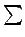(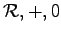)
• Product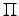, (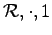)
• Exist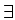, (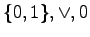)
• All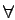, (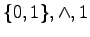)
• Union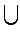, (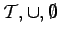)
• Intersection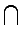, (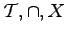)
• Maximum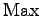, (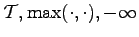)
• Minimum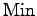, (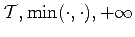)

The union and the intersection operation show that the operations are not necessarily numerical. Indeed, a structurewhich is a topology on a set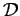fulfills the requirements of a semigroup. It is also possible to characterize the minimum as well as a maximum of a function via this mechanism. It can be seen easily that the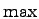function, which returns the maximum of two given arguments is commutative as well as associative. Furthermore an arbitrary neutral element is given so that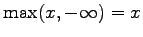and due to the commutativity. Analogously, the minimum function can be introduced, where the neutral element is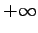.

When traversal functions are used, the results are presented as sets. When using a computer, these sets are given as a certain sequence that covers additional information of the order of the elements. In order to eliminate the influence of the ordering of the elements, only accumulation associative and commutative accumulation methods are allowable. Commutative monoids exactly provide these features and the results are (at least with exact numerics) independent of the special order.

In addition, the number of topological elements within a traversal set can be directly written as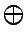. The result of counting the elements is independent from the order of the elements.

An alternative accumulation method is to form a vector from the given elements. It is clear that such a method strongly depends on the order of the elements. In some cases, however, such a formulation can be of favor, especially, if only properties of a vector or a matrix are required, which are invariant with respect to permutations of the lines, e.g., the absolute value of the determinant. The symbol, which is used to denote this vectorization is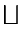.

Michael 2008-01-16